# Clock Puzzles

What is Puzzle

Puzzle is a problem that test imagination, cleverness and skill of the solver. Puzzles are often contrived as a form of Entertainment, But they can also be considered as mathematical or logical problems in most of cases.

People with a high inductive reasoning aptitude may be better in solving those puzzles. puzzles may be solved by good deduction skills.

Clock Puzzles

A clock is an instrument which displays time divided into hours, minutes and seconds.

A clock mainly consists of four components.

Dial

A clock is a circular dial. The periphery of the dial is numbered 1 through 12 indicating the hours in a 12 h cycle.
The circumference of a dial is divided into 60 equal spaces called minute space or 12 equal spaces called hour spaces.

Every clock has mainly two hands, one is smaller and other is bigger. The smaller hand is slower the and the bigger hand is faster.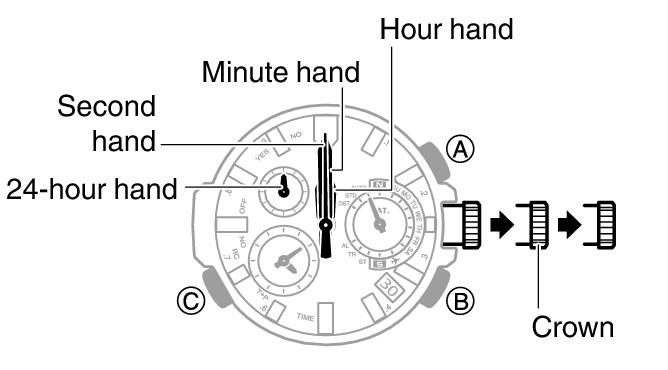1. Minute Spaces:

The face or dial of watch is a circle whose circumference is divided into 60 equal parts, called minute spaces.

2. Hour Hand and Minute Hand:

A clock has two hands; the smaller one is called the hour hand or short hand while the larger one is called minute hand or long hand.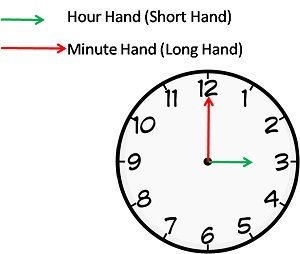3. Second Hand:

Second hand indicates seconds on circular dial. It makes one revolution per minute.

Note : In 1 h minute hand covers 60 min spaces whereas the hour hand cover 5 min spaces.

Therefore, minute hand gains (60 -5) = 55 min in 1 h.

# Important Points Related to Clock:

i. In 60 minutes, the minute hand gains 55 minutes on the hour on the hour hand.

ii. In every hour, both the hands coincide once. (i.e., 0° apart)

For example

Between 11 and 1'o clock, hands are together as shown in adjacent figure.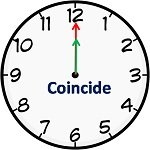iii. The hands are in the same straight line when they are coincident or opposite to each other.

i.e., If two hands are in opposite direction. (180° apart), then they are 30 min spaces apart. This happens once in 1 h. In a period of 12 h both hands are in opposite direction 11 times and in a day both hands are in opposite direction 22 times.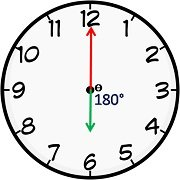iv. When the two hands are at right angles, they are 15 minute spaces apart.

This happens twice in 1 h. In a period of 12 h, the hands are at right angle 22 times (2 common positions) and in a day both hands are at right angle 44 times.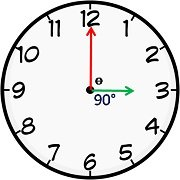v. When the hands are in opposite directions, they are 30 minute spaces apart.

vi. Angle traced by hour hand in 12 hrs = 360°

vii. Angle traced by minute hand in 60 min. = 360°.

viii. If a watch or a clock indicates 8.15, when the correct time is 8, it is said to be 15 minutes too fast.

On the other hand, if it indicates 7.45, when the correct time is 8, it is said to be 15 minutes too slow.

ix. If the real time is given and its MIRROR image is asked or vice – versa, simply subtract the given time from 11:60.

For example the time is 10:10 in the mirror then subtracting it from 11:60 we get 1:50 which will be the mirror image of the clock.

This is a very useful and time saving shortcut for exam and is rarely available in the books which increases its importance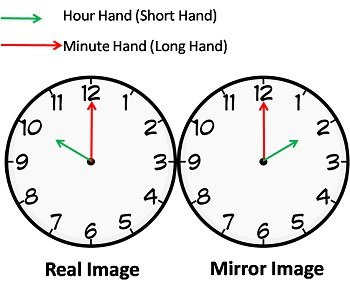x. A broken clock is right twice a day. However, a clock which gains or loses a few minutes might not be right twice a day or even once a day. It would be right when it had gained / lost exactly 12 hours.

Note :

Clock puzzles have specific set of rules, you first has to figure out the pattern being followed and then answer the puzzle according to the pattern.

Clock puzzles are a part of many competitive examinations, these also helps you improve your logical thinking and above all these are fun to crack !!!

HOW TO SOLVE PUZZLES

In general to solve puzzles logical reasoning is necessary. In logic, there are three kinds of logical reasoning. they are

1.deduction
2.induction
3.abduction

precondition and rule implies a conclusion. so based on precondition, induction, abduction we can define above three as below.

DEDUCTION means determining the conclusion. It is using precondition and rule to make conclusion. Mathematicians are commonly used this type of logical reasoning.

INDUCTION means determining the rule. It is learning the rule after numerous examples of conclusion following the precondition. scientists are commonly used this type of logical reasoning.

ABDUCTION means determining the precondition.

It is using conclusion and rule to support that precondition could explain the conclusion. diagnosticians and detectives commonly used this type of logical reasoning.

MIND IT !

To solve puzzles logical reasoning is necessary. With out reasoning we can’t solve any type of logical problems. solving logical puzzles can also improves IQ level and maturity level.

In this section you can practice Clock puzzles and improve your skills in order to face the Interview, Competitive examination and various entrance test with full confidence.